# What are the rules of Magic Squares

 To the main page "Mathematical Handicrafts"

What is a magic square?
That is supposed to be the magic square on Albrecht Dürer's copperplate engraving melancholy to be declared by 1514.

 ...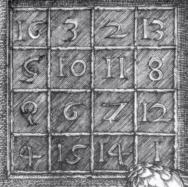.... The numbers 1 to 16 are arranged in a square so that the sum of the numbers below each other, next to each other or diagonally is 34.If you use 1 to 16 as the numbers like here, you get that normal magic square.

Magic squares and variants have long been a popular topic in entertainment mathematics. From the abundance of offers in literature and the Internet, I have put together what I find interesting.

Nth order magic squares Top
The existence of magic squares is certain for all natural numbers n> 2. But there is no known formation law for arbitrary numbers n. - These are examples of the smallest magic squares.

The length of the side also means order. So the examples are magic squares of orders 3, 4, 5, 6, 7 and 8.

Generally one defines:
An nth order square is magical when the numbers 1, 2, 3, ..., n² are distributed in an n x n square in such a way that the sums of the n numbers are constant among each other, next to each other or diagonally. The sum is called the magic number.
The following applies (1 + 2 + 3 + ... + n²): n = (1/2) n (n² + 1).
And that's the magic sums of the first eight squares.
 Magic square:Magic sum: 3x315 4x434 5x565 6x6111 7x7175 8x8260 9x9369 10x10505

The magical 3x3 square
The following applies: 1 + 2 + 3 + 4 + 5 + 6 + 7 + 8 + 9 = 45. In the magic square, 3 numbers are added each time. So the mean sum of three numbers is 45: 3 = 15.
You can also get the magic number 15 if you add the middle summand 5 three times.

The number 15 can be broken down eight times into a sum of three summands:

 15=1+5+915=1+6+8 15=2+4+915=2+5+8 15=2+6+715=3+4+8 15=3+5+715=4+5+6
In the decompositions, the odd numbers 1, 3, 7 and 9 appear twice, the even numbers 2, 4, 6 and 8 appear three times, and the number 5 appears four times.
It follows that you have to put the number 5 in the middle of a magic 3x3 square. The remaining odd numbers have to be placed in the middle of the sides and the even numbers in the corners.
There are eight ways to form a square under these conditions: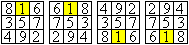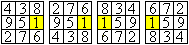...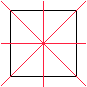... All eight squares merge into one another when they are mirrored about their axes of symmetry.These are the diagonals and the center lines.Symmetrical squares of this type are only counted once.

There is only one magical 3x3 square from this point of view.

Variants of the 3x3 squares Top
Non-normal magic squares

 ...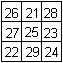... The numbers 21 to 29 form a magic square with the magic sum 75.

The explanation is simple.
 ........ 6 + c .... 1 + c .... 8 + c ................ 7 + c .... 5 + c .... 3 + c ................ 2 + c .... 9 + c .... 4 + c ........ A magic square remains magical if you change every number with a constant c, for example by adding c to every number. Above is c = 20.You can also subtract, multiply or divide.

Square of prime numbers
 ...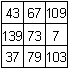... This is a magic square of prime numbers ........................................... ......................................

Square of square numbers
 ...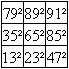... This is a "semi" magic square made up of square numbers.Only the sums through the center are equal .......................................... ......................
Source: http://www.mathpages.com/home/kmath417.htm

The magic multiplication square
This is a magic square where not the sums, but the products of the numbers in the rows, columns and diagonals are the same.
There is a simple rule of education.
 ...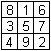... ...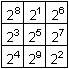... For example, you start from the magic 3x3 square and replace the numbers with powers of two with them as exponents.The magic product is 215=32768.

 ...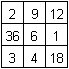... This 3x3 square is the square with the smallest product.The magic product is 216.

The magical 4x4 square Top
Decompositions of the magic number
The magic number is (1 + 2 + ... + 15 + 16): 4 = 34
The computer found 86 decompositions of 34 into a sum of four summands from the numbers 1 to 16:

 34=01+02+15+16 34=01+03+14+1634=01+04+13+16 34=01+04+14+15 34=01+05+12+16 34=01+05+13+15 34=01+06+11+1634=01+06+12+15 34=01+06+13+14 34=01+07+10+16 34=01+07+11+1534=01+07+12+1434=01+08+09+16 34=01+08+10+15 34=01+08+11+14 34=01+08+12+13 34=01+09+10+14 34=01+09+11+13 34=01+10+11+12 34=02+03+13+16 34=02+03+14+15 34=02+04+12+16 34=02+04+13+15 34=02+05+11+16 34=02+05+12+15 34=02+05+13+14 34=02+06+10+1634=02+06+11+15 34=02+06+12+14 34=02+07+09+1634=02+07+10+15 34=02+07+11+14 34=02+07+12+13 34=02+08+09+15 34=02+08+10+14 34=02+08+11+13 34=02+09+10+13 34=02+09+11+1234=03+04+11+16 34=03+04+12+15 34=03+04+13+1434=03+05+10+16 34=03+05+11+15 34=03+05+12+14 34=03+06+09+16 34=03+06+10+15 34=03+06+11+14 34=03+06+12+13 34=03+07+08+16 34=03+07+09+15 34=03+07+10+14 34=03+07+11+13 34=03+08+09+14 34=03+08+10+13 34=03+08+11+12 34=03+09+10+12 34=04+05+09+16 34=04+05+10+15 34=04+05+11+14 34=04+05+12+13 34=04+06+08+16 34=04+06+09+15 34=04+06+10+14 34=04+06+11+13 34=04+07+08+15 34=04+07+09+1434=04+07+10+13 34=04+07+11+12 34=04+08+09+13 34=04+08+10+12 34=04+09+10+11 34=05+06+07+16 34=05+06+08+15 34=05+06+09+14 34=05+06+10+13 34=05+06+11+12 34=05+07+08+14 34=05+07+09+13 34=05+07+10+12 34=05+08+09+12 34=05+08+10+11 34=06+07+08+13 34=06+07+09+12 34=06+07+10+1134=06+08+09+11 34=07+08+09+10 ....
In the decompositions of 34, the summands 1 to 16 are roughly evenly distributed.
 Summand:Number: 0119 0220 0321 0422 0522 0623 0723 0822 0922 1023 1123 1222 1322 1421 1520 1619
In contrast to the 3x3 square, you can hardly draw any conclusions about the distribution of the numbers 1 to 16 in the 4x4 square.

Pattern in Dürer's square
 ...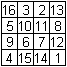.... As mentioned above, Dürer's magic square is simply the magic square in German.

 ...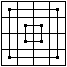... If you mark four numbers with the sum 34, they are often symmetrically distributed in the square and form simple figures like the squares on the left.

In book (7) there are 28 figures that are symmetrical and also symmetrical with respect to the axes of the square.
I was wondering what patterns make up the 86 decompositions of the number 34.
For this purpose, the 86 breakdowns are graphically displayed with the aid of a computer.
If you order the figures, there are, for example, four classes.
default
 ...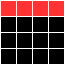.. First of all, there are the 28 symmetrical figures from above from book (7) ...................................

Eightfold
 ...... A figure appears 8 times. .................................................. .................................................. ...................

Fourfold
 ...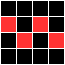... Eight figures appear 4x. With one exception, they are symmetrical .............................That's a total of 32 figures.

Double
 ...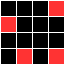...... 9 figures appear twice. They are asymmetrical.That is a total of 18 figures ............................................. .................................................. ............................
Control: 28 + 8 + 32 + 18 = 86

You can find out more about arranging these figures on Harvey Heinz's website Order 4 Magic Squares
(Url below).

Construction of a 4x4 square
There is an easy way to create a magical 4x4 square.

 ...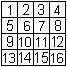............. > Write the numbers from 1 to 16 line by line in the square.

 ...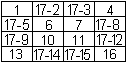... > Leave the numbers in the corners and in the middle 2x2 square.Replace the remaining numbers n with 17-n.

 ...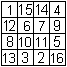... A magical square was created .....
Source: (1) page 134

Axisymmetric magic squares
You get new squares by reflecting on the four axes of the square.
 ......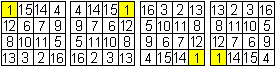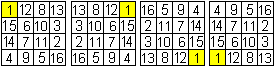As with the magic 3x3 square, the eight variants are seen as the same or equivalent.

It is known that there are a total of 880 magic 4x4 squares.
The axis- and point-symmetrical squares just presented are only counted once.

Point symmetrical magic square
 ...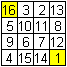... If the two numbers that are point-symmetrical to one another at the center form the sum n² + 1 = 17, then the magic square is called point-symmetrical or self-complementary.The replacement of all numbers n by 17-n means a rotation by 180 °.
The Dürer's square is point-symmetrical.

Semi-magic square
A number square is called semi-magic if only the sum of the numbers below and next to each other is the magic number. One can easily change Dürer's square so that the sum of the numbers on the diagonals is no longer 34.
 ...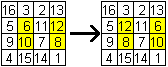... To do this, you swap two pairs lying one below the other with the same sum as on the left. The numbers 6 and 10 are on diagonals and disturb the sums. 34 and 34 become 30 and 38.

Magical parquet
 ...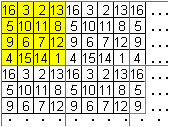... A semi-magical parquet is created when you select any magical square (e.g. Dürer's square) and repeat it next to one another and one below the other.

 ...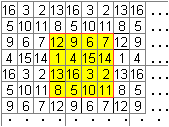... Then every square of 16 numbers is semi-magical .......................................... ...............................

 ...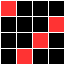... If the parquet-forming square has the additional property that the sums of the "broken" diagonals also have the value of the magic number, it is called panmagic.

 ...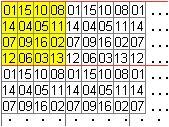... A magical parquet is created when you choose a pan-magical square and repeat it next to each other and one below the other.

 ...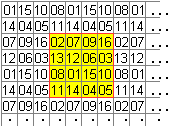... Every square of 16 numbers that you can put in the field is magical ................................... ...

(8), page 253ff.

"Antimagic" square
 ...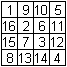... All sums in the rows, in the columns and in the diagonals are different from each other (4).

The magical 5x5 square Top
The magic number is (1 + 2 + ... + 24 + 25) : 5 = 65.

Breakdowns of the magic number 65
 65 = 01+02+13+24+2565 = 01+02+14+23+2565 =  ...65 =  ... 65 =  ...65 =  ...65 = 10+12+13+14+1665 = 11+12+13+14+15 The computer found 1394 decompositions of the number 65.
The summands and their number in the sums: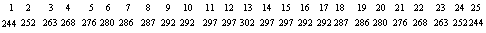It is noticeable that the middle number 13 = 65: 5 is assigned the largest number.
The number of summands for increasing and decreasing summands decreases symmetrically on both sides.

It is known that there are 275 305 224 magic 5x5 squares. (Scientific American 1/1976, German edition)

Construction of a magic 5x5 square
The numbers 1 to 25 are allocated to the fields one after the other. The rules "further up on the right" and "if occupied, continue below" are observed for the next number.
 .....................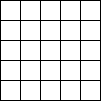.................... The number 1 comes in the middle of the first line.The next number 2 follows in the top right. But then you leave the field.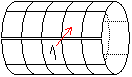Therefore you have to think of the square as a cylinder. The cylinder has the vertical sides as circular circumferences, the horizontal sides meet and close the jacket of the cylinder. Then there is a place for the 2nd in the top right. Unrolled again, the number 2 has moved one field to the right in the last line.
Number 3 follows in the top right.
 ...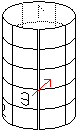... The number 4 would again be outside the field. Imagine a cylinder again, this time with a vertical axis. You can then put the number 4 back in the top right. Unrolled, the number on the third line is on the far left.
The number 5 is on the top right.
A second rule is used for the number 6: If the field at the top right is occupied, the next number goes one line lower in the same column.
One continues with 7.8 and so on. Incidentally, the same rule applies to the number 16 as to the number 6.

This law of formation can be applied to all magic squares with an odd number of pages :-).

Compound squares Top
Frame squares

 ...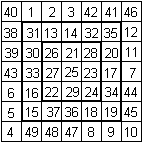... The 7x7 square has the magic number 175.It contains two more magic squares.The 5x5 square has the magic number 125 = 5 * 12 + 65.The 3x3 square has the magic number 75 = 3 * 20 + 15.
(1), page 154

Magic square 9x9 with magic sub-squares
...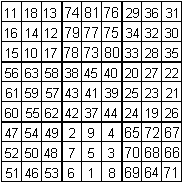...The 9x9 square has the magic number 369.
It is made up of nine magical 3x3 squares.
The magic numbers are
 9+89+549+45 9+729+369+0 9+269+189+63
(1), page 156

Magic square 8x8 with magic sub-squares
 ...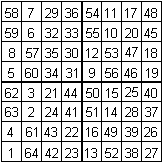... The 8x8 square has the magic number 260.It is made up of four magic 4x4 squares.All four magic squares have the magic number 130.(1), page 155

Special squares Top
Latin square

 ....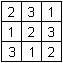.. Here, too, the eight sums in the square are constant.However, only the first three numbers are used.Latin squares are generally used for the nth order.

Magic 21 Square
 ...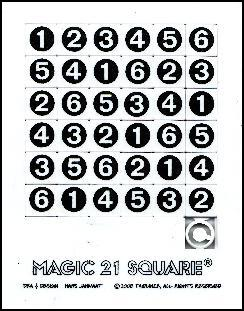... This is a fifteen-piece sliding puzzle.In the five rows and five columns, the sum is 21.The aim of the puzzle is to move the stones so that the total is 21 on the diagonals too.©

Sudoku
 ...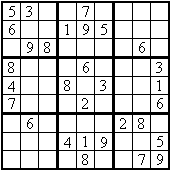...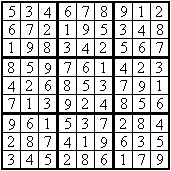... It's a small step from the Latin squares to Sudoku.

Magic letter squares
 ...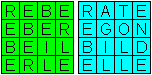... These and similar magic squares made of letters are known from puzzle corners of magazines.The letters form meaningful words.

A well-known square of this type is the sator square, for which there is a Wikipedia page (URL below).

Magic cube Top

 ...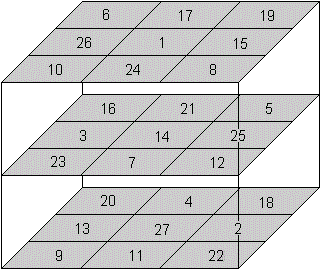... The third order magic cube is a generalization of the 3x3 magic square.The numbers 1 to 27 are distributed on the fields of a 3x3x3 cube in such a way that the sums> the numbers of the 18 lines,> the numbers of the 9 columns> the numbers of the 4 room diagonals are constant.The magic number is 42.
One property has to be dispensed with: The sum of the numbers of the 18 surface diagonals is not 42.

Different point of view
The magic cube contains nine different semi-magic squares.
The following sums lie along the four room diagonals.
 09+14+19=42 22+14+06=42 20+14+08=42 18+14+10=42
(3), page 142f.

No more squares Top
Simple variants with a magic sum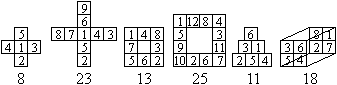Magic rectangle
 ...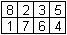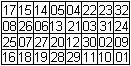The 2x4 rectangle has the magic numbers 9 and 18.The 4x8 rectangle has the magic numbers 66 and 132.(1), page 156

Magical Pythagoras figure
 ...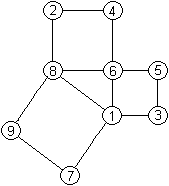... 25² = 20² + 15² can be written as (1 + 8 + 9 + 7) ² = (6 + 4 + 2 + 8) ² + (5 + 3 + 6 + 1) ².This calculation is illustrated by distributing the numbers 1 to 9 on the corners of the Pythagoras figure.
(3), page 143

Magic pentagonal star
 ...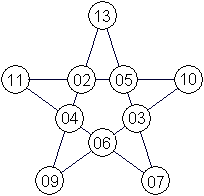... The magic pentagonal star contains ten four-part sums with the magic number 28.10 of the first 13 numbers are used(8), page 213.
Apparently there is no such thing as a normal 5th-order magical star.

Magic hexagon and variants
On my website Magic Hexagon you can find different magic figures in hexagon shape, including magic stars.
Magical heptagonal star
Two normal magic stars with a magic total of 30.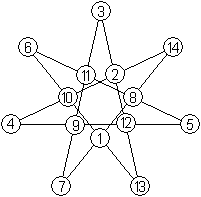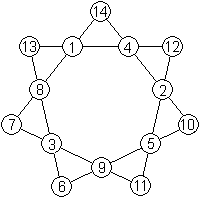Source: Homepage of Harvey D. Heinz (URL below)

 ...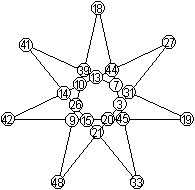Complete magic star21 numbers from the set {3, 4, 5, ..., 46, 47, 48} are used.The magic total is 150.(7) page 24

The magic circle
 ...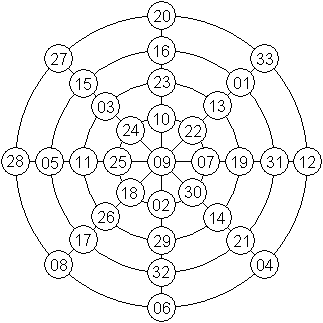... The magic circle contains 4 + 8 = 12 eight-part sums.The sum on each circle is 138.The sum on each diameter (the 9 in the middle must be deleted) is also 138.According to (7) it was designed by the Chinese Yang Hui in 1275.

Magic squares on the internet Top

German

Christoph Pöppe (Spectrum of Science - Dossier)
Noble magic squares

Felix Kunert & Karsten Lehmann (raetselverzeichnis.de)
Magic square puzzle

Gerd Müller
Magic square interactive

Hans-Peter Gramatke
Magic squares

H.B. Meyer
magic squares: 4 x 4, 5 x 5, 6 x 6

Holger Danielsson
Magic squares

Jan Theofel and Martin Trautmann
Magic squares and cubes

Maria Koth
Magic squares (.pdf. file)

Paul Heimbach
Magic squares

recordholders.org
The largest magic square in the world

Udo Hebisch (Mathematical Café)
Magic squares

Wikipedia
Magic Square, Perfectly Perfect Magic Square, Sator Square, Magic Cube,
Latin square, witches' uniqueness

English

Craig Knecht (Magic Square Models)

 ...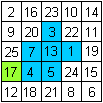... Imagine: The squares are the tips of square prisms at the height of the numbers. If you pour water on this body, it remains in the middle up to height 17 as a pond. There it flows away. The amount of water is (17-3) + (17-7) + (17-13) + (17-1) + (17-4) + (17-5) = 69.There are nice problems: Largest amount of water? Separate lakes? Islands?Water retention patterns

 ...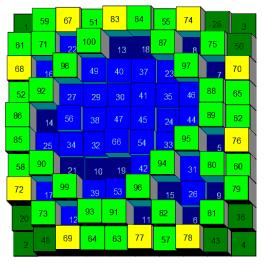... The next picture clearly shows what is under Water retention patterns is to be understood.You can find programs for this on the website http://users.eastlink.ca/~sharrywhite/Download.html(no longer online)

Eric W. Weisstein (MathWorld)
Magic Square, Panmagic Square, Associative Magic Square, Lo Shu, Prime Magic Square, Magic Cube, Magic Circles

Harvey D. Heinz
Magic Hypercubes Home Page, Order 4 Magic Squares

H.B. Meyer
magic squares: 4 x 4, 5 x 5, 6 x 6

Holger Danielsson
Magic Squares

Mark S. Farrar
Magic Squares

MathPages
Solving Magic Squares

Robin Moseley
Magic Flexagon (.pdf file)

The On-Line Encyclopedia of Integer Sequences
magic numbers, sequences related to ..., Sequence of magic numbers: A006003

Wikipedia
Magic square, Most-perfect magic square, Sator Square, Magic cube, Magic star, Simple magic cube,
Latin square, Magic circle (mathematics), Water retention on mathematical surfaces, Associative magic square

credentials Top
(1) Hermann Schubert: Mathematical leisure hours, Berlin 1941 (first edition)
(2) Wilhelm Ahrens: Mathematical entertainments and games, Leipzig 1901
(3) Walter Lietzmann: Funny and strange things about numbers and shapes, Göttingen 1969
(4) Bild der Wissenschaft, 8/1966, 6/1968, 7/1971, 9/1971, 3/1974, 10/1976
(5) Pieter van Delft / Jack Botermans: Puzzle of the World, Munich 1980 (reissued in 1998)
(6) Maximilian Miller: Solved and unsolved mathematical problems, Leipzig 1982
(7) Kenneth Kelsey: Magic Numbers Games, dtv 1983 [ISBN 3-423-10199-7]
(8) Jan Gullberg: Mathematics - From the Birth of Numbers, New York - London 1997 (ISBN0-393-04002-X)
(9) Tibor Bakos: Magical Squares, in "Mathematisches Mosaik", Cologne 1977 [ISBN 3-7614-0371-2]

Feedback: Email address on my main page

The page, which was revised in 2011, is not available in English.

URL of my homepage:
http://www.mathematische-basteleien.de/

© 2000 Jürgen Köller

Top